### Sluice Gate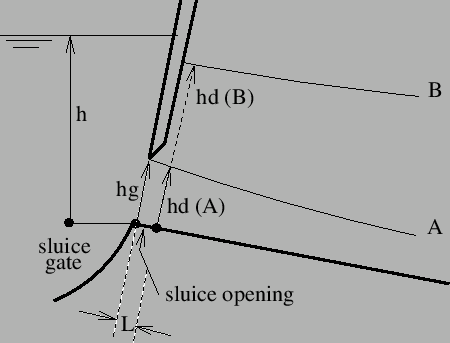The sluice gate is the upstream element of a pair of boundary elements simulating a sluice. The downstream element is the sluice opening. Both are illustrated in Figure 120. The interesting point is that the gate height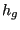may be part of the backwater curve, but it does not have to. If the lower point of the gate is higher than the fluid surface, it will not be part of the backwater curve.

If the gate door touches the water and the water curve is a frontwater curve (curve A in Figure 120) the volumetric flow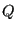is given by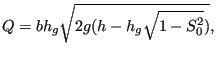(161)

if the gate door does not touch the water and the water curve is a frontwater curve the volumetric flowis given by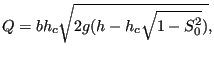(162)

where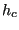is the critical depth. If the gate door touches the water and the water curve is a backwater curve (governed by downstream boundary conditions, curve B in Figure 120)) the volumetric flow is given by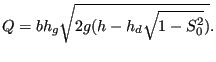(163)

Finally, if the gate door does not touch the water and the water curve is a backwater curve the volumetric flow is given by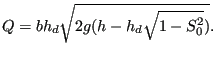(164)

The following constants have to be specified on the line beneath the *FLUID SECTION,TYPE=CHANNEL SLUICE GATE card:

• the width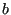• the slope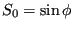(if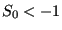the slope is calculated from the coordinates of the end nodes belonging to the element)
• the height of the gate door• not used
• the number of the downstream sluice opening element
• the number of the upstream reference element, if any

The slope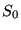is used in case the gate door does not touch the water surface. The cross section of a sluice gate is supposed to be rectangular. Therefore,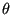is lacking in its definition. Notice that a sluice gate can have upstream channel elements attached to it. In that case it is mandatory to specify an upstream reference element.

Example files: channel1, chanson1.